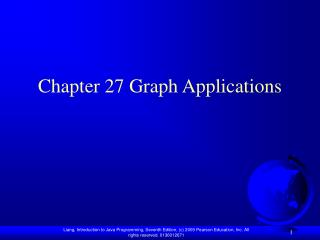Download PresentationChapter 27 Graph Applications

# Chapter 27 Graph Applications - PowerPoint PPT PresentationDownload Presentation## Chapter 27 Graph Applications

- - - - - - - - - - - - - - - - - - - - - - - - - - - E N D - - - - - - - - - - - - - - - - - - - - - - - - - - -
##### Presentation Transcript

1. Chapter 27 Graph Applications

2. Objectives • To give applications of graphs and explain the Seven Bridges of Königsberg problem (§27.1). • To describe the graph terminologies: vertices, edges, simple graphs, weighted/unweighted graphs, and directed/undirected graphs (§27.2). • To represent vertices and edges using lists, adjacent matrices, and adjacent lists (§27.3). • To model graphs using the Graph interface, the AbstractGraph class, and the UnweightedGraph class (§27.4). • To represent the traversal of a graph using the AbstractGraph.Tree class (§27.5). • To design and implement depth-first search (§27.6). • To design and implement breadth-first search (§27.7). • To solve the nine tail problem using breadth-first search (§27.8).

3. Modeling Using Graphs

4. Seven Bridges of Königsberg

5. Basic Graph Terminologies What is a graph? Define a graph Directed vs. undirected graphs Weighted vs. unweighted graphs Adjacent vertices Incident Degree Neighbor loop

6. Basic Graph Terminologies Parallel edge Simple graph Complete graph Spanning tree

7. Representing Graphs Representing Vertices Representing Edges: Edge Array Representing Edges: Edge Objects Representing Edges: Adjacency Matrices Representing Edges: Adjacency Lists

8. Modeling Graphs

9. Graph AbstractGraph UnweightedGraph TestGraph

10. Graph Traversals Depth-first search and breadth-first search Both traversals result in a spanning tree, which can be modeled using a class.

11. Depth-First Search The depth-first search of a graph is like the depth-first search of a tree discussed in §25.2.3, “Tree Traversal.” In the case of a tree, the search starts from the root. In a graph, the search can start from any vertex. dfs(vertex v) { visit v; for each neighbor w of v if (w has not been visited) { dfs(w); } }

12. Depth-First Search Example

13. Depth-First Search Example TestDFS TestDFS

14. Applications of the DFS Detecting whether a graph is connected. A graph is connected if there is a path between any two vertices in the graph. (See Exercise 27.1) Detecting whether there is a path between two vertices. (See Exercise 27.2) Finding a path between two vertices. (See Exercise 27.3) Finding all connected components. A connected component is a maximal connected subgraph in which every pair of vertices are connected by a path. (See Exercise 27.4) Detecting whether there is a cycle in the graph. (See Exercise 27.5) Finding a cycle in the graph. (See Exercise 27.5)

15. Breadth-First Search The breadth-first traversal of a graph is like the breadth-first traversal of a tree discussed in §25.2.3, “Tree Traversal.” With breadth-first traversal of a tree, the nodes are visited level by level. First the root is visited, then all the children of the root, then the grandchildren of the root from left to right, and so on.

16. Breadth-First Search Algorithm bfs(vertex v) { create an empty queue for storing vertices to be visited; add v into the queue; mark v visited; while the queue is not empty { dequeue a vertex, say u, from the queue visit u; for each neighbor w of u if w has not been visited { add w into the queue; mark w visited; } } }

18. Breadth-First Search Example TestBFS TestBFS

19. Applications of the BFS Detecting whether a graph is connected. A graph is connected if there is a path between any two vertices in the graph. Detecting whether there is a path between two vertices. Finding a shortest path between two vertices. You can prove that the path between the root and any node in the BFS tree is the shortest path between the root and the node (see Review Question 27.10). Finding all connected components. A connected component is a maximal connected subgraph in which every pair of vertices are connected by a path. Detecting whether there is a cycle in the graph. (See Exercise 27.4) Finding a cycle in the graph. (See Exercise 27.5) Testing whether a graph is bipartite. A graph is bipartite if the vertices of the graph can be divided into two disjoint sets such that no edges exist between vertices in the same set. (See Exercise 27.8)

20. The Nine Tail Problem The problem is stated as follows. Nine coins are placed in a three by three matrix with some face up and some face down. A legal move is to take any coin that is face up and reverse it, together with the coins adjacent to it (this does not include coins that are diagonally adjacent). Your task is to find the minimum number of the moves that lead to all coins face down.

21. The Nine Tail Problem NineTailModel NineTailApp NineTailApp

22. The Hamiltonian Path Problem A Hamiltonian path in a graph is a path that visits each vertex in the graph exactly once. A Hamiltonian cycle is a cycle that visits each vertex in the graph exactly once and returns to the starting vertex. A Hamiltonian path and cycle have many applications.

23. The Knight’s Tour Problem The Knight’s Tour is a well-known classic problem. The objective is to move a knight, starting from any square on a chessboard, to every other square once. Note that the knight makes only L-shape moves (two spaces in one direction and one space in a perpendicular direction). As shown in Figure 27.19(a), the knight can move to eight squares.

24. Find a Knight’s Tour To solve the Knight’s Tour problem, create a graph with 64 vertices representing all the squares in the chessboard. Two vertices are connected if a knight can move between the two vertices. KnightTourModel KnightTourApp KnightTourApp